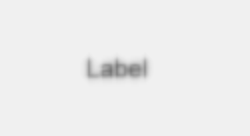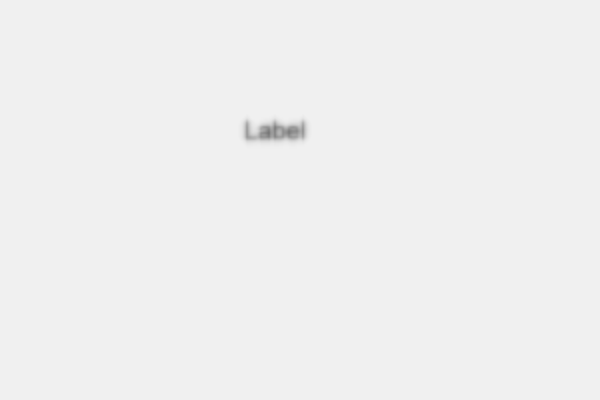# PyQt5 QLabel – Adding blur effect

• Last Updated : 10 May, 2020

In this article we will see how we can add blur effect to the label, blur means smoothing of the label, by default there is no blur effect to the label although we can add blur effect any time. Below is the representation of label with blur effect.In order to do this we have to do the following –

1. Create a label
2. Create QGraphicsBlurEffect object
3. Add this object to the label with the help of setGraphicsEffect method

Syntax :

```# creating a blur effect
self.blur_effect = QGraphicsBlurEffect()

# adding blur effect to the label
label.setGraphicsEffect(self.blur_effect)
```

Below is the implementation

 `# importing libraries``from` `PyQt5.QtWidgets ``import` `*` `from` `PyQt5 ``import` `QtCore, QtGui``from` `PyQt5.QtGui ``import` `*` `from` `PyQt5.QtCore ``import` `*` `import` `sys`` ` ` ` `class` `Window(QMainWindow):`` ` `    ``def` `__init__(``self``):``        ``super``().__init__()`` ` `        ``# setting title``        ``self``.setWindowTitle(``"Python "``)`` ` `        ``# setting geometry``        ``self``.setGeometry(``100``, ``100``, ``600``, ``400``)`` ` `        ``# calling method``        ``self``.UiComponents()`` ` `        ``# showing all the widgets``        ``self``.show()`` ` `    ``# method for widgets``    ``def` `UiComponents(``self``):`` ` `        ``# creating label``        ``label ``=` `QLabel(``"Label"``, ``self``)`` ` `        ``# setting geometry to the label``        ``label.setGeometry(``200``, ``100``, ``150``, ``60``)`` ` `        ``# setting alignment to the label``        ``label.setAlignment(Qt.AlignCenter)`` ` `        ``# setting font``        ``label.setFont(QFont(``'Arial'``, ``15``))`` ` `        ``# creating a blur effect``        ``self``.blur_effect ``=` `QGraphicsBlurEffect()`` ` `        ``# adding blur effect to the label``        ``label.setGraphicsEffect(``self``.blur_effect)`` ` ` ` `# create pyqt5 app``App ``=` `QApplication(sys.argv)`` ` `# create the instance of our Window``window ``=` `Window()`` ` `# start the app``sys.exit(App.``exec``())`

Output :My Personal Notes arrow_drop_up